# DC Source Conversion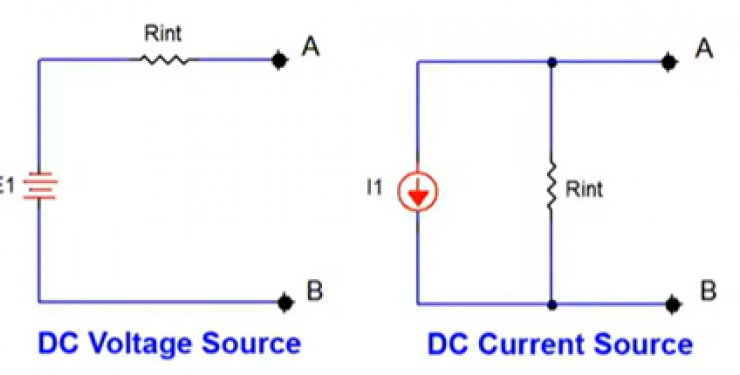In this article we will be reviewing DC source conversion and understand how it can be useful when analyzing circuits. DC sources are mainly referred to as sources of constant voltages or currents. Hence, we can classify them into two types; DC Voltage Source and DC Current Source, where each source type representation includes an internal resistance “Rint” as illustrated  in the figure below. We will analyze how easy it is to convert between these two source types while maintaining the same internal resistance, Rint.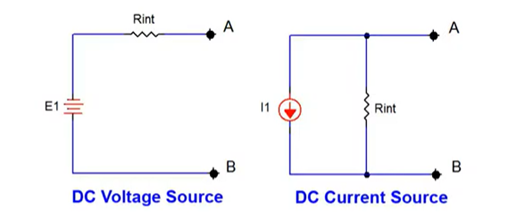### The Purpose of Conversion

You might wonder what’s the need for conversion? Well, the ability to convert between the two source types can be extremely useful when performing Nodal Analysis or Loop Analysis as well as when attempting to create Thevenin and/or Norton equivalent circuits.

Current Source –  Let’s create the equivalent Current Source for a 12V voltage source having an internal resistance of 75 ohms.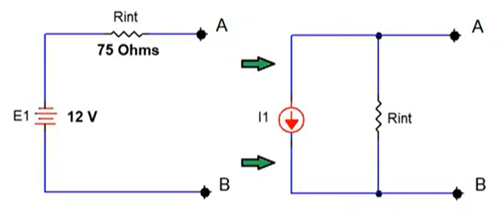As our intention is for both sources to contain the same internal resistance Rint, we will use the same resistor value as we configure our current source circuit, and these circuits will behave identically when a load is connected to them. As we proceed, calculating the current source value is simply done by applying basic Ohm's law: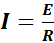. The appropriate source current value is derived by dividing the voltage by the internal resistance as shown below:

IS=E1Rint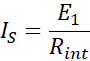IS=12V75 Ohms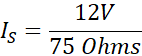IS=160mA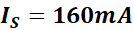We now have two circuits that will behave identically with respect to various loads connected to points A and B as shown in the figure below.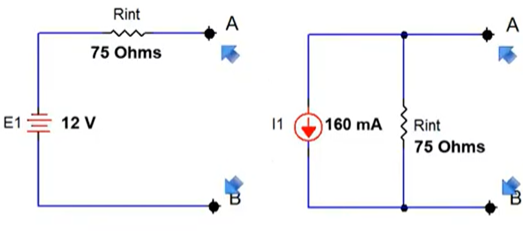Now, let us move on to perform the same type of conversion in reverse.

Voltage Source – Let us now create the equivalent Voltage Source for a 50mA current source having an internal resistance of 300 ohms.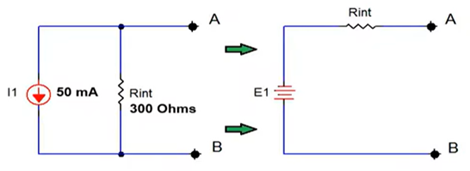Both sources will contain the same internal resistance value, Rint = 300 Ohms. Therefore, calculating the voltage source value is simply done by applying basic Ohm's law: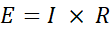. The required voltage source value is a derived by multiplying the current by the internal resistance as shown below:

E1=I × Rint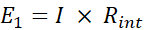E1=50mA × 300 Ohms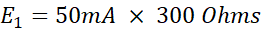E1=15Volts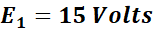Here too we now have two circuits that will behave identically with respect to various loads connected to points A and B as shown in the figure below: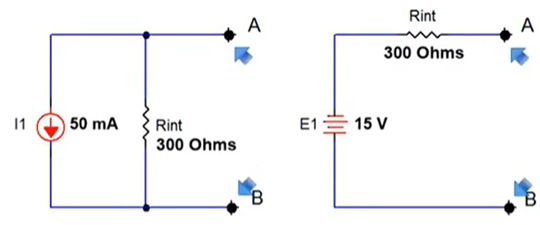In concluding, we recognise that source conversion is quite easy and it is a great tool when you're using DC linear Network theorems for circuit reduction purposes.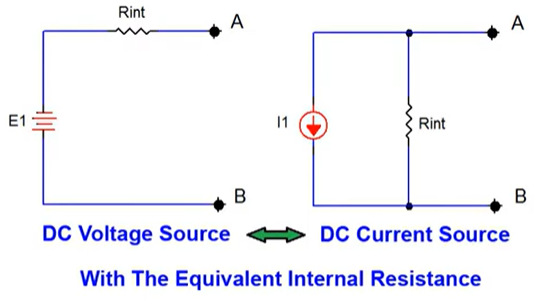We hope that this has been helpful as a student or practicing Electronics technician. If you have any questions regarding the Electronics Technician program, feel free to get in touch with us at info@gbctechtraining.com or give us a call at 1-888-553-5333 to speak with a Program Consultant.